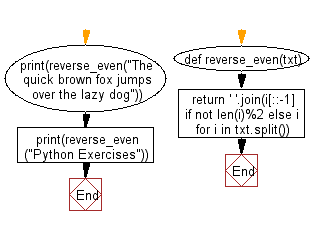﻿ Python: Reverse all the words which have even length - w3resource# Python: Reverse all the words which have even length

## Python Basic - 1: Exercise-113 with Solution

Write a Python program to reverse all the words which have even length.

Sample Solution:

Python Code:

``````def reverse_even(txt):
return ' '.join(i[::-1] if not len(i)%2 else i for i in txt.split())
print(reverse_even("The quick brown fox jumps over the lazy dog"))
print(reverse_even("Python Exercises"))
``````

Sample Output:

```The quick brown fox jumps revo the yzal dog
nohtyP Exercises
```

Pictorial Presentation:Flowchart:Python Code Editor:

Have another way to solve this solution? Contribute your code (and comments) through Disqus.

What is the difficulty level of this exercise?

Test your Programming skills with w3resource's quiz.

﻿

## Python: Tips of the Day

What is the difference between Python's list methods append and extend?

append: Appends object at the end.

```x = [1, 2, 3]
x.append([4, 5])
print (x)
```

Output:

```[1, 2, 3, [4, 5]]
```

extend: Extends list by appending elements from the iterable.

```x = [1, 2, 3]
x.extend([4, 5])
print (x)
```

Output:

```[1, 2, 3, 4, 5]
```

Ref: https://bit.ly/2AZ6ZFq